# How to Average the Last N Values in Excel

In Excel there are a lot of built-in functions, and AVERAGE is one of the most frequently used functions. We can apply it to calculate the average of numbers from a given range reference. In daily work, we may apply AVERAGE function together with some other functions to get average based on some conditions. For example, get the average of sales in the nearest 3 days. In this article, we will let you know the method to apply AVERAGE function together with OFFSET function and COUNT function to get the average of last N values from a dynamic range.

In this article, we will introduce you the syntax, arguments, and basic usage of above three functions. We will also explain how the formula works with these functions.

## EXAMPLE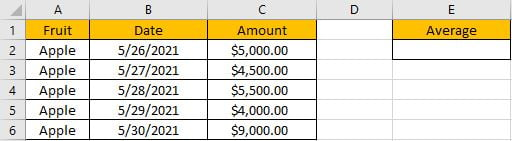In this case, we want to calculate the average of the last 3 values in “Amount” column. If “Amount” range is a fixed range, we can directly create formula “=AVERAGE(C4:C6)” to get the average of last 3 values. If this range is a dynamic range, for example add a new line for date 5/31/2021, the last 3 values are changed due to a new row is inserted. So, the simple formula with only AVERAGE function doesn’t work.

To get the average of last N values for a dynamic range, we can apply AVERAGE function together with other functions to help us. In this article, to approach our goal, we also apply OFFSET function together with COUNT function to help us set our average range.

## FORMULA with AVERAGE & OFFSET & COUNT FUNCTIONS

In E2, enter the formula =AVERAGE(OFFSET(C1,COUNT(C:C),0,-3)), then press Enter, average of last three amounts is \$6166.67.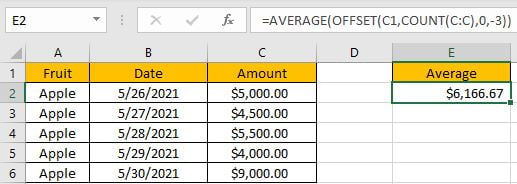(5500+4000+9000)/3=6166.666666, keep two decimal places 6166.67. The formula works correctly.

## FUNCTION INTRODUCTION

AVERAGE function returns the average of numbers from a given range reference.

Syntax:

`=AVERAGE(number1, [number2], …)`

Usage: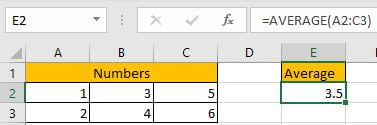OFFSET returns a number or a dynamic range based on given five parameters: starting point, row offset, column offset, height in rows and width in rows.

Syntax:

`=OFFSET(reference, rows, cols, [height], [width])`

Usage 1: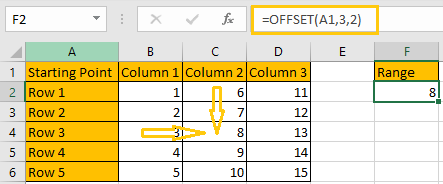Reference: A1, it is the starting point

Rows: 3, 3 rows below the starting point

Cols: 2, 2 columns to offset to the right of the starting point

Height & Width: optional, no value

Usage 2: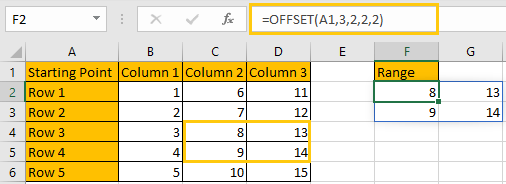Reference: A1, it is the starting point

Rows: 3, 3 rows below the starting point

Cols: 2, 2 columns to offset to the right of the starting point

Height: 2, it is used together with width, determine the size of the returned range reference, in this case is 2*2 (two rows*two columns)

Width: 2, it is used together with height, determine the size of the returned range reference, in this case is 2*2 (two rows*two columns)

COUNT returns the count of numbers from a given range.

Syntax:

` =COUNT(value1, [value2], …)`

Usage: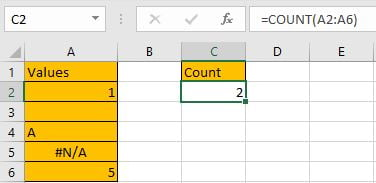## EXPLANATION

`=AVERAGE(OFFSET(C1,COUNT(C:C),0,-3))`

In this formula, C:C is a full column reference, it represents the entire C column; if user want to cover entire C, D and E columns, we can enter C:E to represent multiple columns, just enter the start column index and end column index, use colon “:” to concentrate them. A full row reference is similar with a full column reference, for example, 1:3 means the first three entire rows. We often use full column or row reference when the selected range is a dynamic range, and user may add or delete column or row at any moment.

In this case, COUNT(C:C) returns 5, there are five cells with numbers in column C.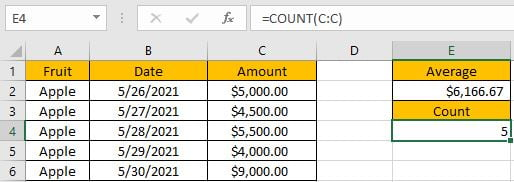Now, for OFFSET part, the five inputs are:

Reference: C1 (header of column C, it will not be included when counting row and columns)

Rows: 5. 5 rows below the starting point. The returned range is started in row 6.

Cols: 0. No column to offset to the starting point.

Based on above “Rows” and “Cols” two parameters, we can confirm one point of our returned range, it is C6.Height: -3. It represents that the returned range is 3 rows in height. Currently, Excel OFFSET function can handle the case height and width are negative numbers. In this case, height value is “-3”, so from C6, OFFSET function expands the range 3 rows backwards to the starting point C1.

Width: No value. Width value is optional for OFFSET function. If it is omitted, the returned range is only limited in current column.

Based on above “Height” and “Width”, we can confirm the returned range, it is C4:C6.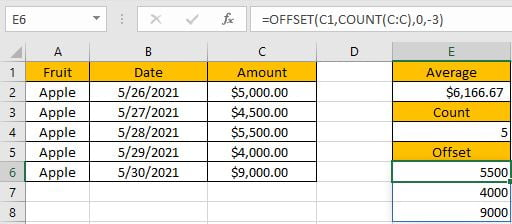Now, =AVERAGE(OFFSET(C1,COUNT(C:C),0,-3)) is equal to =AVERAGE(C4:C6). After above steps, we get correct value 6166.67.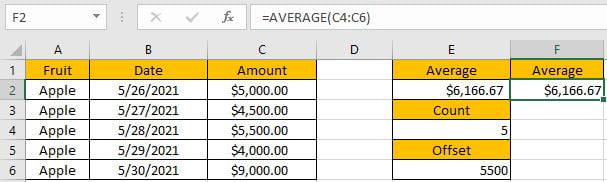SUMMARY

1. AVERAGE function is used for returning the average of some numbers from a given range in Excel.

2. OFFSET function returns a number or a dynamic range based on given five inputs. Actually, rows, cols, height and width can be input negative numbers.

3. COUNT function only returns count of numbers.

### Related Functions

• Excel AVERAGE function
The Excel AVERAGE function returns the average of the numbers that you provided.The syntax of the AVERAGE function is as below:=AVERAGE (number1,[number2],…)….
• Excel COUNT function
The Excel COUNT function counts the number of cells that contain numbers, and counts numbers within the list of arguments. It returns a numeric value that indicate the number of cells that contain numbers in a range…
Related Posts

Calculate Grades With VLookup in Excel

Why Should You Calculate Grades With VLookup Excel? If you're looking for a simple way to find out a student's grades, VLOOKUP Excel can do the trick. This function uses a lookup table to find the values and sort them ...

Repeating Character n Times in Excel

In daily life, we can use repeated characters to indicate the magnitude or priority of something. For example, a single “!” indicates a minor case, double “!!” indicates a medium case, and triple “!!!” indicates a high case, and so ...

Calculate Cumulative Loan Interest in Excel

What is Cumulative Loan Interest? When comparing different types of loans, many people want to know what is Cumulative Loan Interest. The sum of all interest payments you've made on a loan is referred to as cumulative interest. Different lenders ...

Calculate Cumulative Loan Principal Payments in Excel

How do you Calculate Cumulative Loan Principal Payments in Excel? There are a few different ways to calculate this information, but one way has been proven to be accurate more often than others. One option is to use the CUMPRINC ...

Trap Error or Replace Error by Specific Value with IFERROR function

We often use Excel formulas in our work life, and we may encounter this situation that the formula throws an error and finally an error like #DIV/0! Is displayed in the cell. In today’s tutorial, we will introduce you the ...

Calculate Compound Interest in Excel

This article will show you how to calculate compound interest in Excel. It will help you calculate the interest you will accrue on a given amount of money. There are several ways to calculate compound interest. One of the simplest ...

Count Attendance and Absence with COUNTIF function

In our campus life and work life, we usually record everyone's attendance. Today we will introduce you the application of Excel COUNTIF function to count the attendance. Using a week as an example, we use the following example to show ...

Find the Closest Data to the Data Provided in Excel

In our daily work, we may encounter such an issue that to find the closest value to a certain value. In fact, Excel internal functions can help us solve this problem. In today’s article, we will show you how to ...

Average Of Numbers With Multiple Criteria In Excel

Have you ever come across a task to calculate the average of the numbers with respect to multiple criteria? Are you tired of doing this cumbersome task manually? Are you willing to do this task smartly in just a matter ...

Calculate Average Of Last 5 Or N Values In Columns

Suppose you come across a task where you need to calculate the average of the last 2 or 3 numeric values, then what would you do? If you are new to Excel, then your first attempt might be doing this ...

Sidebar